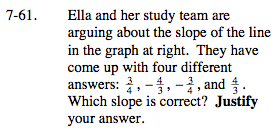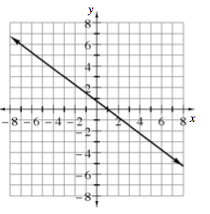### Home > MC2 > Chapter Ch7 > Lesson 7.1.6 > Problem7-61

7-61.$\text{Remember that the slope can be written as \frac{change\; in\; {\it y}}{change\; in\; {\it x}}.}$

On this line as the x-value increases, does the y-value decrease or increase?
Decreasing makes the slope negative.
Increasing makes the slope positive.

Try answering this problem by going from the point (−3, 3) to the point (1, 0).